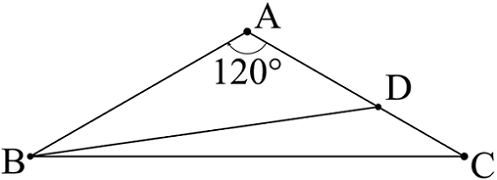# Prime TriangleThe figure above shows an isosceles triangle $ABC$ with $BA = AC$ and $\angle BAC = 120^\circ$.

Let $D$ be a point on $AC$ such that $AD > DC$. Given that the lengths of $BA$, $AD$, $DC$, and $BD$ are all distinct prime numbers, what is the length of $BD?$

×